Publicité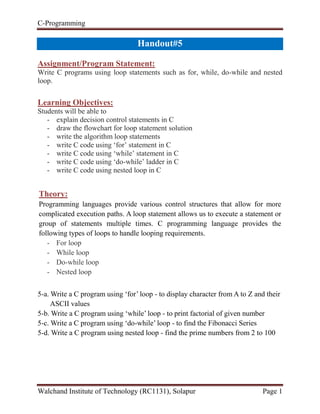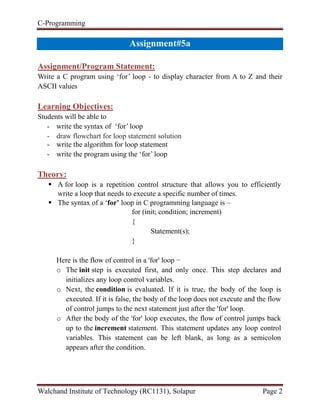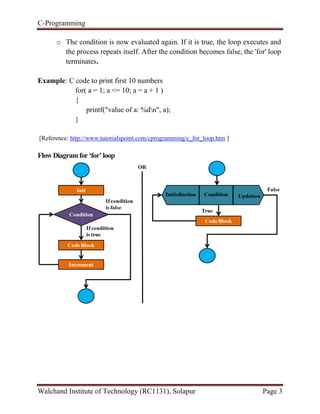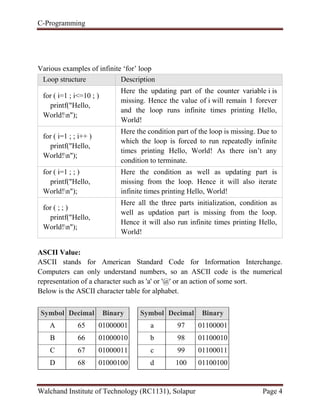Publicité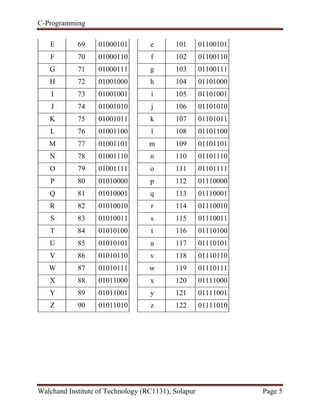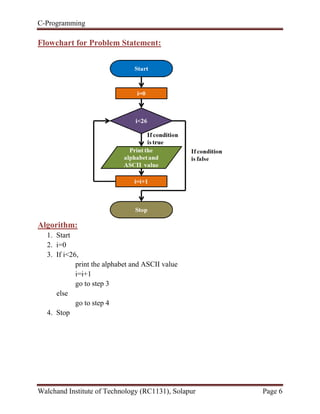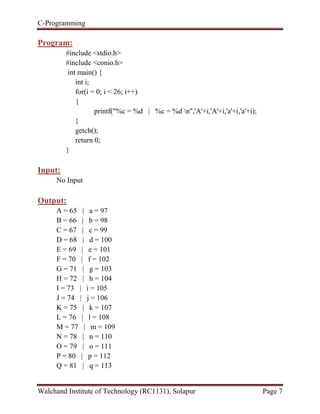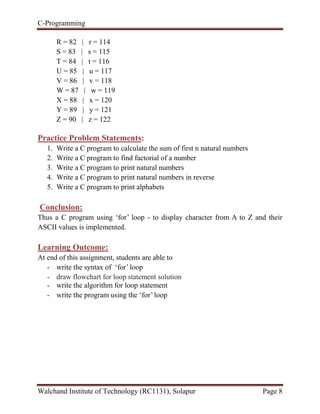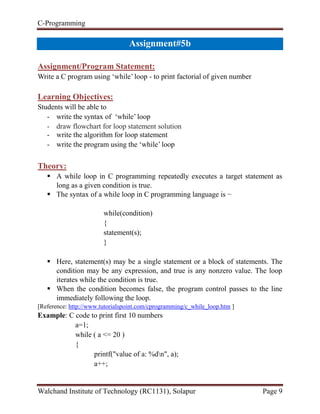Publicité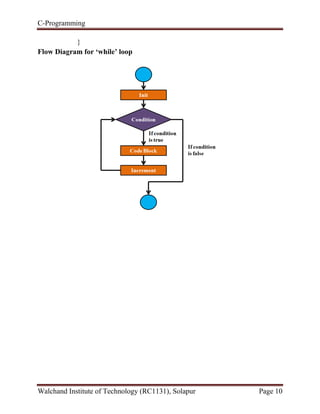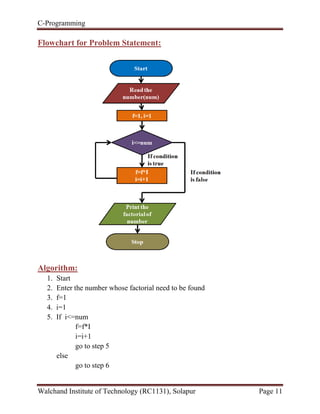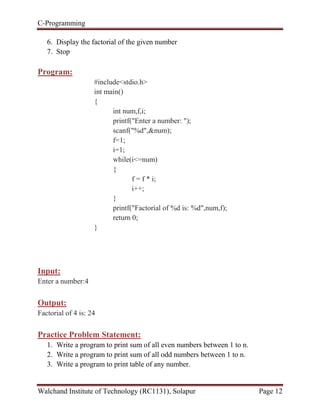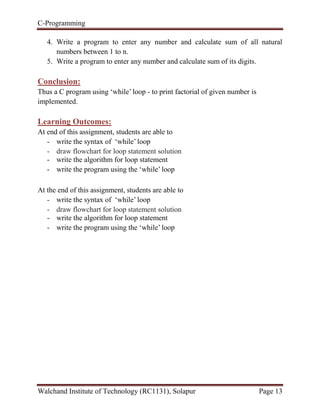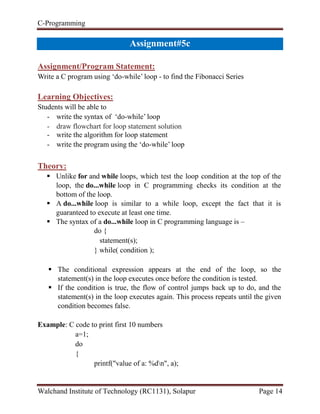Publicité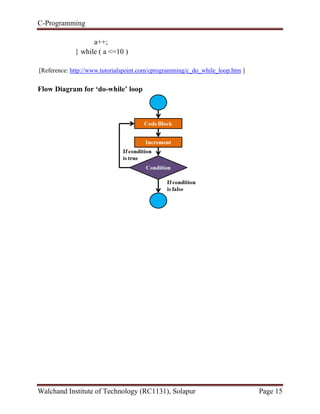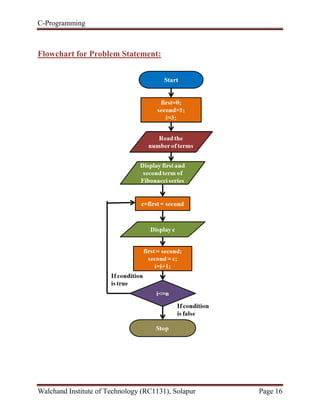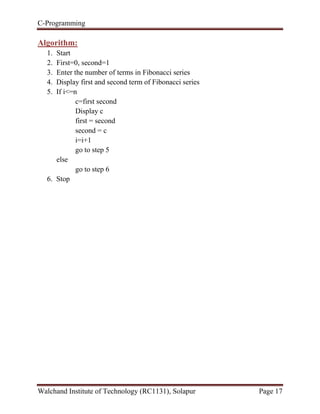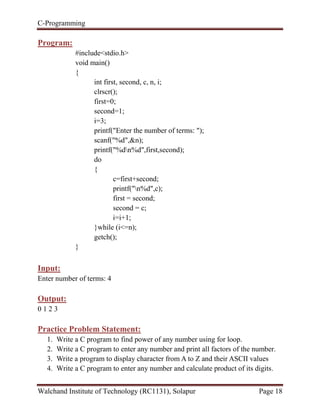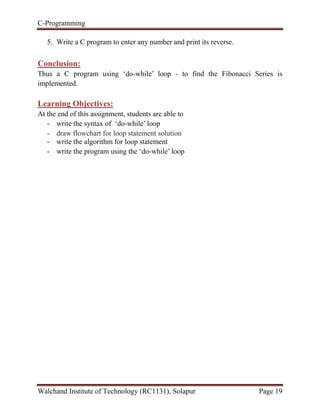Publicité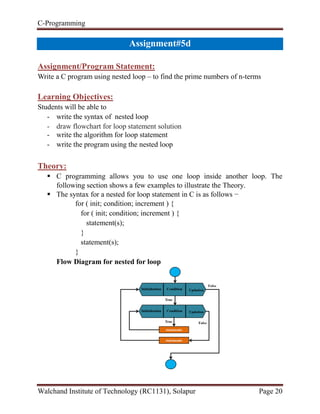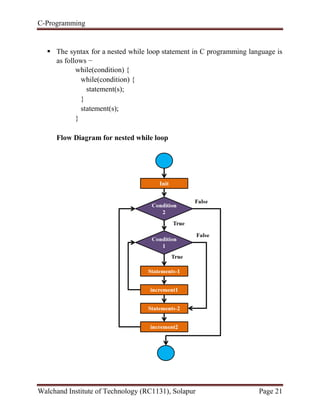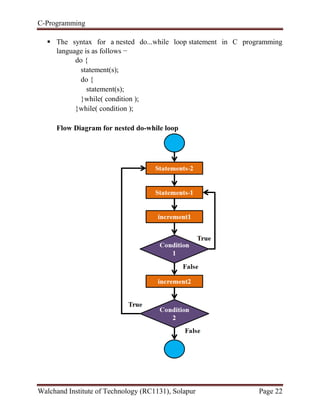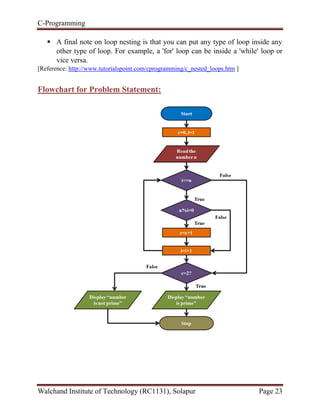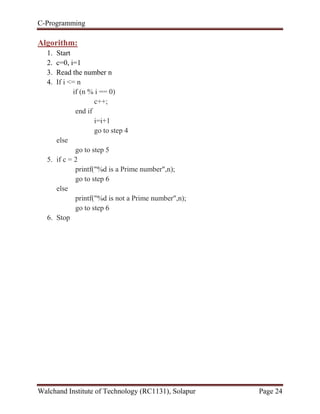Publicité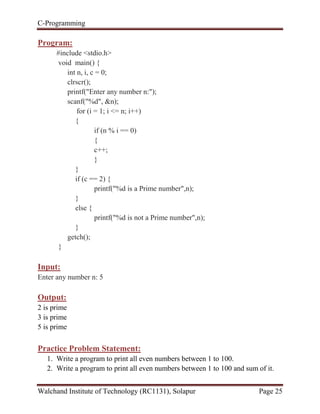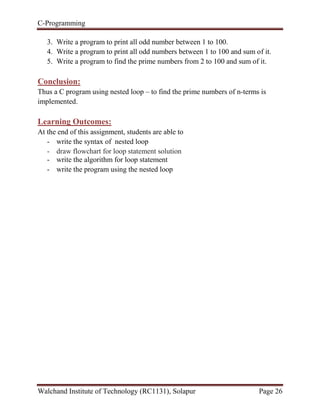Prochain SlideShare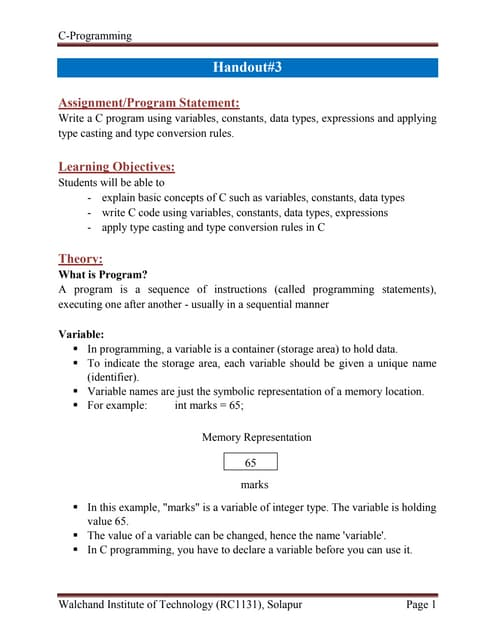CP Handout#3
Chargement dans ... 3
1 sur 26
Publicité

### CP Handout#5

1. C-Programming Walchand Institute of Technology (RC1131), Solapur Page 1 Handout#5 Assignment/Program Statement: Write C programs using loop statements such as for, while, do-while and nested loop. Learning Objectives: Students will be able to - explain decision control statements in C - draw the flowchart for loop statement solution - write the algorithm loop statements - write C code using ‘for’ statement in C - write C code using ‘while’ statement in C - write C code using ‘do-while’ ladder in C - write C code using nested loop in C Theory: Programming languages provide various control structures that allow for more complicated execution paths. A loop statement allows us to execute a statement or group of statements multiple times. C programming language provides the following types of loops to handle looping requirements. - For loop - While loop - Do-while loop - Nested loop 5-a. Write a C program using ‘for’ loop - to display character from A to Z and their ASCII values 5-b. Write a C program using ‘while’ loop - to print factorial of given number 5-c. Write a C program using ‘do-while’ loop - to find the Fibonacci Series 5-d. Write a C program using nested loop - find the prime numbers from 2 to 100
2. C-Programming Walchand Institute of Technology (RC1131), Solapur Page 2 Assignment#5a Assignment/Program Statement: Write a C program using ‘for’ loop - to display character from A to Z and their ASCII values Learning Objectives: Students will be able to - write the syntax of ‘for’ loop - draw flowchart for loop statement solution - write the algorithm for loop statement - write the program using the ‘for’ loop Theory:  A for loop is a repetition control structure that allows you to efficiently write a loop that needs to execute a specific number of times.  The syntax of a ‘for’ loop in C programming language is – for (init; condition; increment) { Statement(s); } Here is the flow of control in a 'for' loop − o The init step is executed first, and only once. This step declares and initializes any loop control variables. o Next, the condition is evaluated. If it is true, the body of the loop is executed. If it is false, the body of the loop does not execute and the flow of control jumps to the next statement just after the 'for' loop. o After the body of the 'for' loop executes, the flow of control jumps back up to the increment statement. This statement updates any loop control variables. This statement can be left blank, as long as a semicolon appears after the condition.
3. C-Programming Walchand Institute of Technology (RC1131), Solapur Page 3 o The condition is now evaluated again. If it is true, the loop executes and the process repeats itself. After the condition becomes false, the 'for' loop terminates. Example: C code to print first 10 numbers for( a = 1; a <= 10; a = a + 1 ) { printf("value of a: %dn", a); } [Reference: http://www.tutorialspoint.com/cprogramming/c_for_loop.htm ] Flow Diagramfor‘for’loop
4. C-Programming Walchand Institute of Technology (RC1131), Solapur Page 4 Various examples of infinite ‘for’ loop Loop structure Description for ( i=1 ; i<=10 ; ) printf("Hello, World!n"); Here the updating part of the counter variable i is missing. Hence the value of i will remain 1 forever and the loop runs infinite times printing Hello, World! for ( i=1 ; ; i++ ) printf("Hello, World!n"); Here the condition part of the loop is missing. Due to which the loop is forced to run repeatedly infinite times printing Hello, World! As there isn’t any condition to terminate. for ( i=1 ; ; ) printf("Hello, World!n"); Here the condition as well as updating part is missing from the loop. Hence it will also iterate infinite times printing Hello, World! for ( ; ; ) printf("Hello, World!n"); Here all the three parts initialization, condition as well as updation part is missing from the loop. Hence it will also run infinite times printing Hello, World! ASCII Value: ASCII stands for American Standard Code for Information Interchange. Computers can only understand numbers, so an ASCII code is the numerical representation of a character such as 'a' or '@' or an action of some sort. Below is the ASCII character table for alphabet. Symbol Decimal Binary A 65 01000001 B 66 01000010 C 67 01000011 D 68 01000100 Symbol Decimal Binary a 97 01100001 b 98 01100010 c 99 01100011 d 100 01100100
5. C-Programming Walchand Institute of Technology (RC1131), Solapur Page 5 E 69 01000101 F 70 01000110 G 71 01000111 H 72 01001000 I 73 01001001 J 74 01001010 K 75 01001011 L 76 01001100 M 77 01001101 N 78 01001110 O 79 01001111 P 80 01010000 Q 81 01010001 R 82 01010010 S 83 01010011 T 84 01010100 U 85 01010101 V 86 01010110 W 87 01010111 X 88 01011000 Y 89 01011001 Z 90 01011010 e 101 01100101 f 102 01100110 g 103 01100111 h 104 01101000 i 105 01101001 j 106 01101010 k 107 01101011 l 108 01101100 m 109 01101101 n 110 01101110 o 111 01101111 p 112 01110000 q 113 01110001 r 114 01110010 s 115 01110011 t 116 01110100 u 117 01110101 v 118 01110110 w 119 01110111 x 120 01111000 y 121 01111001 z 122 01111010
6. C-Programming Walchand Institute of Technology (RC1131), Solapur Page 6 Flowchart for Problem Statement: Algorithm: 1. Start 2. i=0 3. If i<26, print the alphabet and ASCII value i=i+1 go to step 3 else go to step 4 4. Stop
7. C-Programming Walchand Institute of Technology (RC1131), Solapur Page 7 Program: #include <stdio.h> #include <conio.h> int main() { int i; for(i = 0; i < 26; i++) { printf("%c = %d | %c = %d n",'A'+i,'A'+i,'a'+i,'a'+i); } getch(); return 0; } Input: No Input Output: A = 65 | a = 97 B = 66 | b = 98 C = 67 | c = 99 D = 68 | d = 100 E = 69 | e = 101 F = 70 | f = 102 G = 71 | g = 103 H = 72 | h = 104 I = 73 | i = 105 J = 74 | j = 106 K = 75 | k = 107 L = 76 | l = 108 M = 77 | m = 109 N = 78 | n = 110 O = 79 | o = 111 P = 80 | p = 112 Q = 81 | q = 113
8. C-Programming Walchand Institute of Technology (RC1131), Solapur Page 8 R = 82 | r = 114 S = 83 | s = 115 T = 84 | t = 116 U = 85 | u = 117 V = 86 | v = 118 W = 87 | w = 119 X = 88 | x = 120 Y = 89 | y = 121 Z = 90 | z = 122 Practice Problem Statements: 1. Write a C program to calculate the sum of first n natural numbers 2. Write a C program to find factorial of a number 3. Write a C program to print natural numbers 4. Write a C program to print natural numbers in reverse 5. Write a C program to print alphabets Conclusion: Thus a C program using ‘for’ loop - to display character from A to Z and their ASCII values is implemented. Learning Outcome: At end of this assignment, students are able to - write the syntax of ‘for’ loop - draw flowchart for loop statement solution - write the algorithm for loop statement - write the program using the ‘for’ loop
9. C-Programming Walchand Institute of Technology (RC1131), Solapur Page 9 Assignment#5b Assignment/Program Statement: Write a C program using ‘while’ loop - to print factorial of given number Learning Objectives: Students will be able to - write the syntax of ‘while’ loop - draw flowchart for loop statement solution - write the algorithm for loop statement - write the program using the ‘while’ loop Theory:  A while loop in C programming repeatedly executes a target statement as long as a given condition is true.  The syntax of a while loop in C programming language is − while(condition) { statement(s); }  Here, statement(s) may be a single statement or a block of statements. The condition may be any expression, and true is any nonzero value. The loop iterates while the condition is true.  When the condition becomes false, the program control passes to the line immediately following the loop. [Reference: http://www.tutorialspoint.com/cprogramming/c_while_loop.htm ] Example: C code to print first 10 numbers a=1; while ( a <= 20 ) { printf("value of a: %dn", a); a++;
10. C-Programming Walchand Institute of Technology (RC1131), Solapur Page 10 } Flow Diagram for ‘while’ loop
11. C-Programming Walchand Institute of Technology (RC1131), Solapur Page 11 Flowchart for Problem Statement: Algorithm: 1. Start 2. Enter the number whose factorial need to be found 3. f=1 4. i=1 5. If i<=num f=f*I i=i+1 go to step 5 else go to step 6
12. C-Programming Walchand Institute of Technology (RC1131), Solapur Page 12 6. Display the factorial of the given number 7. Stop Program: #include<stdio.h> int main() { int num,f,i; printf("Enter a number: "); scanf("%d",&num); f=1; i=1; while(i<=num) { f = f * i; i++; } printf("Factorial of %d is: %d",num,f); return 0; } Input: Enter a number:4 Output: Factorial of 4 is: 24 Practice Problem Statement: 1. Write a program to print sum of all even numbers between 1 to n. 2. Write a program to print sum of all odd numbers between 1 to n. 3. Write a program to print table of any number.
13. C-Programming Walchand Institute of Technology (RC1131), Solapur Page 13 4. Write a program to enter any number and calculate sum of all natural numbers between 1 to n. 5. Write a program to enter any number and calculate sum of its digits. Conclusion: Thus a C program using ‘while’ loop - to print factorial of given number is implemented. Learning Outcomes: At end of this assignment, students are able to - write the syntax of ‘while’ loop - draw flowchart for loop statement solution - write the algorithm for loop statement - write the program using the ‘while’ loop At the end of this assignment, students are able to - write the syntax of ‘while’ loop - draw flowchart for loop statement solution - write the algorithm for loop statement - write the program using the ‘while’ loop
14. C-Programming Walchand Institute of Technology (RC1131), Solapur Page 14 Assignment#5c Assignment/Program Statement: Write a C program using ‘do-while’ loop - to find the Fibonacci Series Learning Objectives: Students will be able to - write the syntax of ‘do-while’ loop - draw flowchart for loop statement solution - write the algorithm for loop statement - write the program using the ‘do-while’ loop Theory:  Unlike for and while loops, which test the loop condition at the top of the loop, the do...while loop in C programming checks its condition at the bottom of the loop.  A do...while loop is similar to a while loop, except the fact that it is guaranteed to execute at least one time.  The syntax of a do...while loop in C programming language is – do { statement(s); } while( condition );  The conditional expression appears at the end of the loop, so the statement(s) in the loop executes once before the condition is tested.  If the condition is true, the flow of control jumps back up to do, and the statement(s) in the loop executes again. This process repeats until the given condition becomes false. Example: C code to print first 10 numbers a=1; do { printf("value of a: %dn", a);
15. C-Programming Walchand Institute of Technology (RC1131), Solapur Page 15 a++; } while ( a <=10 ) [Reference: http://www.tutorialspoint.com/cprogramming/c_do_while_loop.htm ] Flow Diagram for ‘do-while’ loop
16. C-Programming Walchand Institute of Technology (RC1131), Solapur Page 16 Flowchart for Problem Statement:
17. C-Programming Walchand Institute of Technology (RC1131), Solapur Page 17 Algorithm: 1. Start 2. First=0, second=1 3. Enter the number of terms in Fibonacci series 4. Display first and second term of Fibonacci series 5. If i<=n c=first second Display c first = second second = c i=i+1 go to step 5 else go to step 6 6. Stop
18. C-Programming Walchand Institute of Technology (RC1131), Solapur Page 18 Program: #include<stdio.h> void main() { int first, second, c, n, i; clrscr(); first=0; second=1; i=3; printf("Enter the number of terms: "); scanf("%d",&n); printf("%dn%d",first,second); do { c=first+second; printf("n%d",c); first = second; second = c; i=i+1; }while (i<=n); getch(); } Input: Enter number of terms: 4 Output: 0 1 2 3 Practice Problem Statement: 1. Write a C program to find power of any number using for loop. 2. Write a C program to enter any number and print all factors of the number. 3. Write a program to display character from A to Z and their ASCII values 4. Write a C program to enter any number and calculate product of its digits.
19. C-Programming Walchand Institute of Technology (RC1131), Solapur Page 19 5. Write a C program to enter any number and print its reverse. Conclusion: Thus a C program using ‘do-while’ loop - to find the Fibonacci Series is implemented. Learning Objectives: At the end of this assignment, students are able to - write the syntax of ‘do-while’ loop - draw flowchart for loop statement solution - write the algorithm for loop statement - write the program using the ‘do-while’ loop
20. C-Programming Walchand Institute of Technology (RC1131), Solapur Page 20 Assignment#5d Assignment/Program Statement: Write a C program using nested loop – to find the prime numbers of n-terms Learning Objectives: Students will be able to - write the syntax of nested loop - draw flowchart for loop statement solution - write the algorithm for loop statement - write the program using the nested loop Theory:  C programming allows you to use one loop inside another loop. The following section shows a few examples to illustrate the Theory.  The syntax for a nested for loop statement in C is as follows − for ( init; condition; increment ) { for ( init; condition; increment ) { statement(s); } statement(s); } Flow Diagram for nested for loop
21. C-Programming Walchand Institute of Technology (RC1131), Solapur Page 21  The syntax for a nested while loop statement in C programming language is as follows − while(condition) { while(condition) { statement(s); } statement(s); } Flow Diagram for nested while loop
22. C-Programming Walchand Institute of Technology (RC1131), Solapur Page 22  The syntax for a nested do...while loop statement in C programming language is as follows − do { statement(s); do { statement(s); }while( condition ); }while( condition ); Flow Diagram for nested do-while loop
23. C-Programming Walchand Institute of Technology (RC1131), Solapur Page 23  A final note on loop nesting is that you can put any type of loop inside any other type of loop. For example, a 'for' loop can be inside a 'while' loop or vice versa. [Reference: http://www.tutorialspoint.com/cprogramming/c_nested_loops.htm ] Flowchart for Problem Statement:
24. C-Programming Walchand Institute of Technology (RC1131), Solapur Page 24 Algorithm: 1. Start 2. c=0, i=1 3. Read the number n 4. If i <= n if (n % i == 0) c++; end if i=i+1 go to step 4 else go to step 5 5. if c = 2 printf("%d is a Prime number",n); go to step 6 else printf("%d is not a Prime number",n); go to step 6 6. Stop
25. C-Programming Walchand Institute of Technology (RC1131), Solapur Page 25 Program: #include <stdio.h> void main() { int n, i, c = 0; clrscr(); printf("Enter any number n:"); scanf("%d", &n); for (i = 1; i <= n; i++) { if (n % i == 0) { c++; } } if (c == 2) { printf("%d is a Prime number",n); } else { printf("%d is not a Prime number",n); } getch(); } Input: Enter any number n: 5 Output: 2 is prime 3 is prime 5 is prime Practice Problem Statement: 1. Write a program to print all even numbers between 1 to 100. 2. Write a program to print all even numbers between 1 to 100 and sum of it.
26. C-Programming Walchand Institute of Technology (RC1131), Solapur Page 26 3. Write a program to print all odd number between 1 to 100. 4. Write a program to print all odd numbers between 1 to 100 and sum of it. 5. Write a program to find the prime numbers from 2 to 100 and sum of it. Conclusion: Thus a C program using nested loop – to find the prime numbers of n-terms is implemented. Learning Outcomes: At the end of this assignment, students are able to - write the syntax of nested loop - draw flowchart for loop statement solution - write the algorithm for loop statement - write the program using the nested loop
Publicité